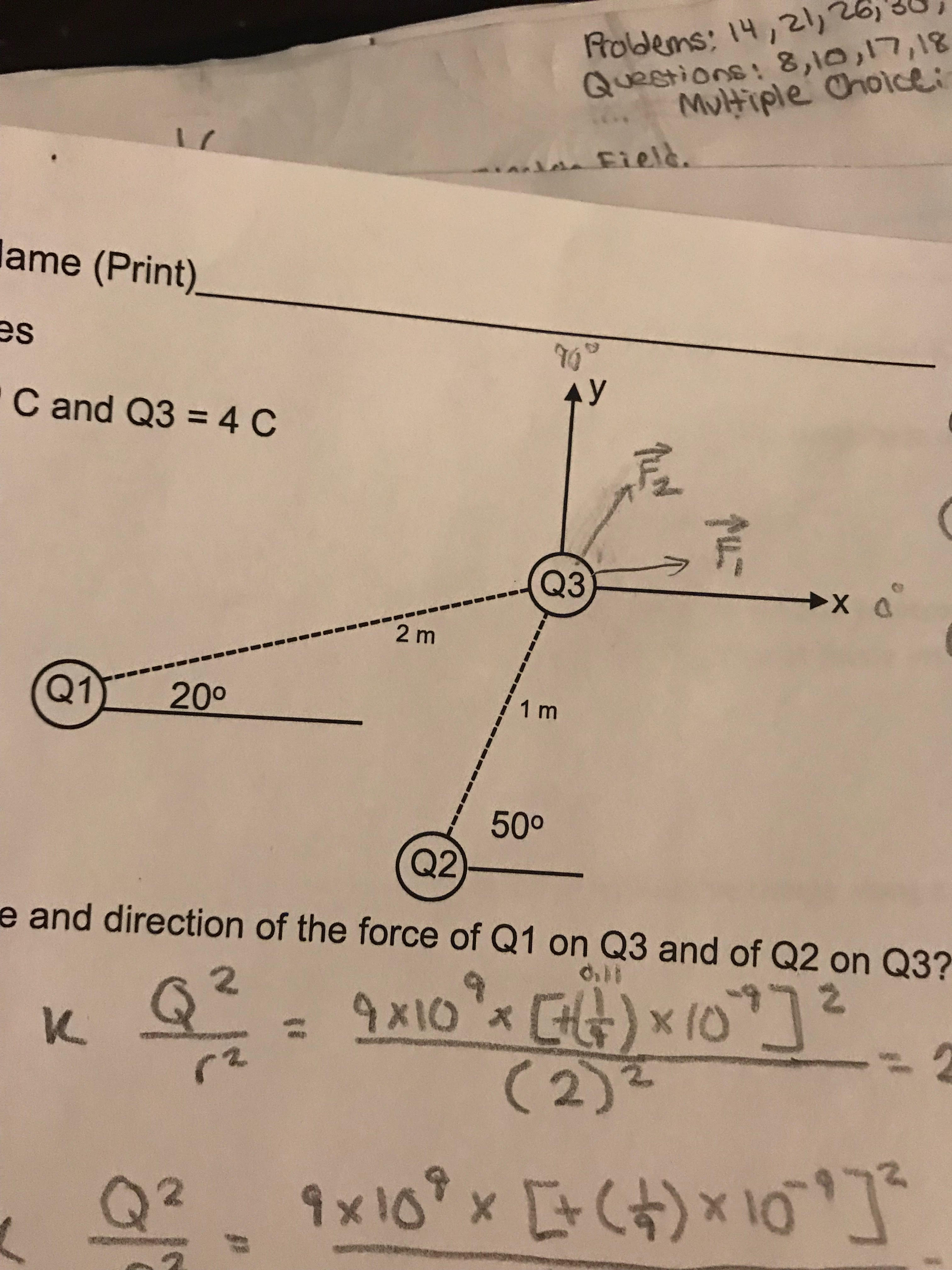# 35,Rodens:14,21)26)Questions: 8,l0,17,18Muttiple onoiceiField.lame (Print)es"GサC and Q34 CQ32 mQ11 2001 m500Q2)-e and direction of the force of Q1 on Q3 and of Q2 on Q3?(2)

Question
8 views

You are given 3 charges Q1=Q2= +(1/9)x10^-9 C and Q3 = 4 C
Image attached of the set up

1. What is the magnitude and direction of the force of Q1 on Q3 and of Q2 on Q3?

2. What is the magnitude and direction of the net force on Q3?help_outlineImage Transcriptionclose35, Rodens:14,21)26) Questions: 8,l0,17,18 Muttiple onoicei Field. lame (Print) es "Gサ C and Q3 4 C Q3 2 m Q11 200 1 m 500 Q2)- e and direction of the force of Q1 on Q3 and of Q2 on Q3? (2) fullscreen
check_circle

Step 1

According to coulomb's law , electric force by a charge on another charge is given as

Step 2

Consider charge Q1 and Q3 and assume the distance between them as r1 such that

Step 3

Hence the force by charge Q1 on Q3  is given as

hence force by charge Q1 on Q3 is...

### Want to see the full answer?

See Solution

#### Want to see this answer and more?

Solutions are written by subject experts who are available 24/7. Questions are typically answered within 1 hour.*

See Solution
*Response times may vary by subject and question.
Tagged in

### Physics